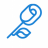# Using MTF InertiaAll in ThinkScript

F

#### flyer01

##### New member
Has anyone had luck with the InertiaAll function for a higher timeframe? For example, before entering a trade, I like to make sure the trend on both the one hour and four hour time frame are moving in the same direction (per InertiaAll). However, when using the script below on a one hour chart to show the straight line trend over four hours, it clearly isn't correct. Any ideas on how to use InertiaAll over multiple time frames?

Code:
``````declare lower;

plot inert = InertiaAll(data = close(period = AggregationPeriod.FOUR_HOURS), length = 100);``````

T

#### tomsk

##### Well-known member
VIP
Has anyone had luck with the InertiaAll function for a higher timeframe? For example, before entering a trade, I like to make sure the trend on both the one hour and four hour time frame are moving in the same direction (per InertiaAll). However, when using the script below on a one hour chart to show the straight line trend over four hours, it clearly isn't correct. Any ideas on how to use InertiaAll over multiple time frames?

Code:
``````declare lower;

plot inert = InertiaAll(data = close(period = AggregationPeriod.FOUR_HOURS), length = 100);``````

@flyer01 I typically have used Inertiaall() on upper studies in the past. I took your code base and was able to plot it just fine on a 1 hour chart, using a 4 Hour secondary aggregation. Here's the code

Code:
``````input length = 100;
plot LR = InertiaAll(close(period = AggregationPeriod.FOUR_HOURS), length);
LR.setDefaultColor(Color.Cyan);
def dist = AbsValue(LR - close);
plot Upper = LR + HighestAll(dist);
plot Lower = LR - HighestAll(dist);``````

F

#### flyer01

##### New member
@tomsk I am getting similar results with the code in post #1 and post #2. I think the issue is it's using a length of 100 on the base chart. For example, if I have a one hour chart displayed, the four hour aggregation is based on 100 one hour bars instead of 100 four hour bars.

Today (Sunday 12/1), the code in post #2 on /ES has a midline value of 3076.75 100 bars ago on the 4 hour chart. When switching to a 1 hour chart, the beginning midline value is 3129.86. Since the aggregation period 4 hours, I would expect the beginning midline value to always be 3076.75.

Edit: added the word "beginning" in italics.

T

#### tomsk

##### Well-known member
VIP
Just make sure you have sufficient bars on your charts.
On a 20 day 4 hour chart I have 78 bars while on 20 day 1 hour I have 212 bars
I personally don't use MTF studies.

•markos#### Daedalus77

##### New member
I'm tinkering with the InertiaAll function for the first time today. Does anyone know how to get it to extend the lines to the end of the chart (e.g. 50 bars into the future)?

Here's the code from ToS Learning Center (slightly modified)

Code:
``````declare upper;
input price = close;
input length = 200;

plot MiddleLR = InertiaAll(price,length,no,yes);
def dist = HighestAll(AbsValue(MiddleLR - price));
plot UpperLR = MiddleLR + dist;
plot LowerLR = MiddleLR - dist;``````#### Daedalus77

##### New member
Disregard that. I wasn't specifying the start date and time.Scanner is using older candles than what is intended Questions 0